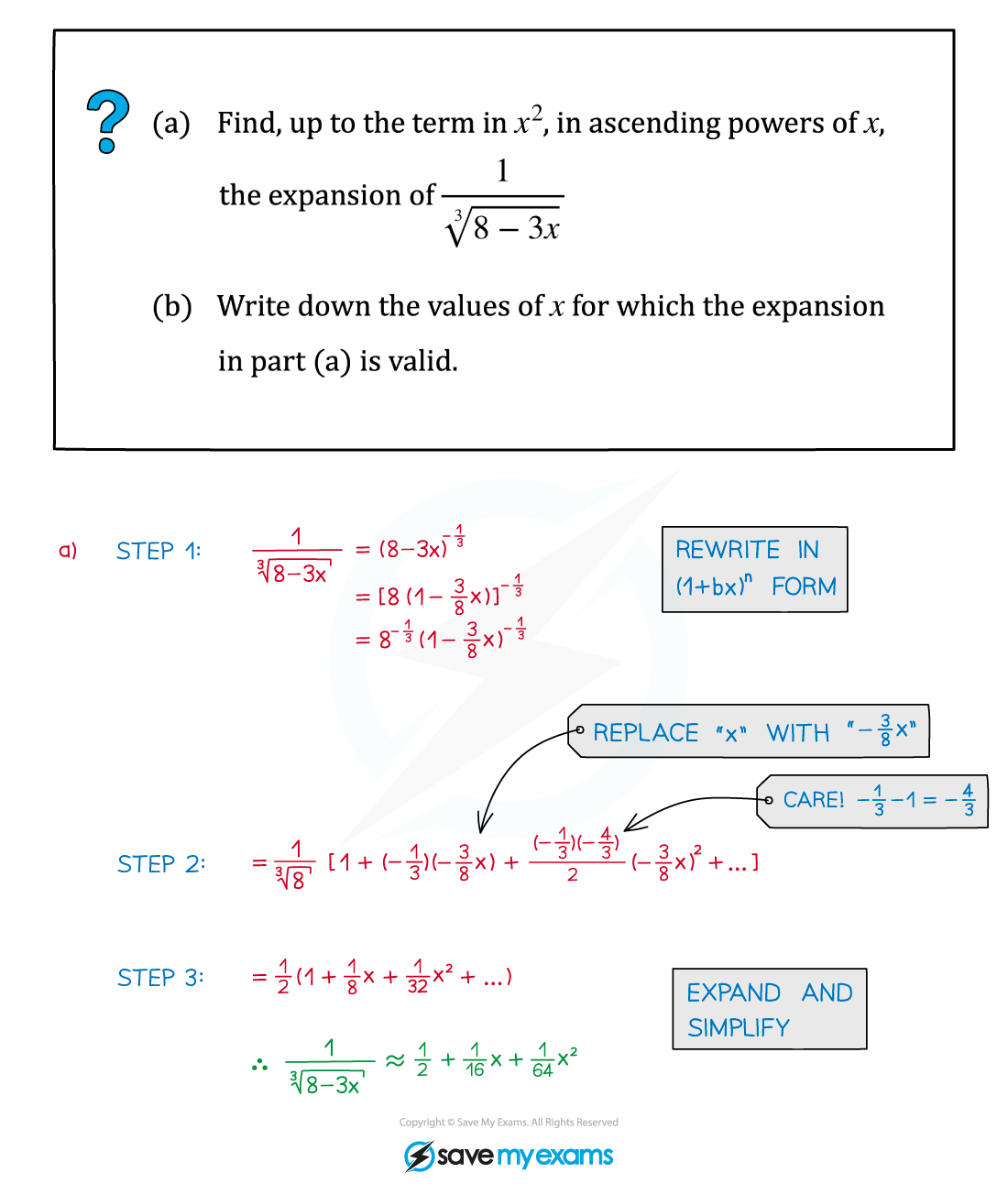# AQA A Level Maths: Pure复习笔记4.2.2 General Binomial Expansion - Subtleties

### General Binomial Expansion - Subtleties

#### What is the general binomial expansion?

• The binomial expansion applies for positive integers, n ∈ ℕ
• The general binomial expansion applies to other types of powers too• The general binomial expansion applies for all real numbers, n ∈ℝ
• Usually fractional and/or negative values of n are used
• It is derived from (a + b)n, with a = 1 and b = x
• Even when a ≠ 1 the general binomial expansion can still be used

#### How do I use the general binomial expansion for (a + kx)n?

The general binomial expansion can be applied to expanding (a + kx)n

STEP 1        Rewrite the question into (1 + bx)n form

• Roots are fractional powers
• Denominators are negative powers
• A factor may be needed to make “a = 1”

STEP 2        Replace “x” by “bx” in the expansion

Check carefully to see if b is negative

STEP 3        Expand and simplify

Use a line for each term to make things easier to read and follow

Use brackets

STEP 4        If required, check and state the validity statement, |bx| < 1#### Worked Example